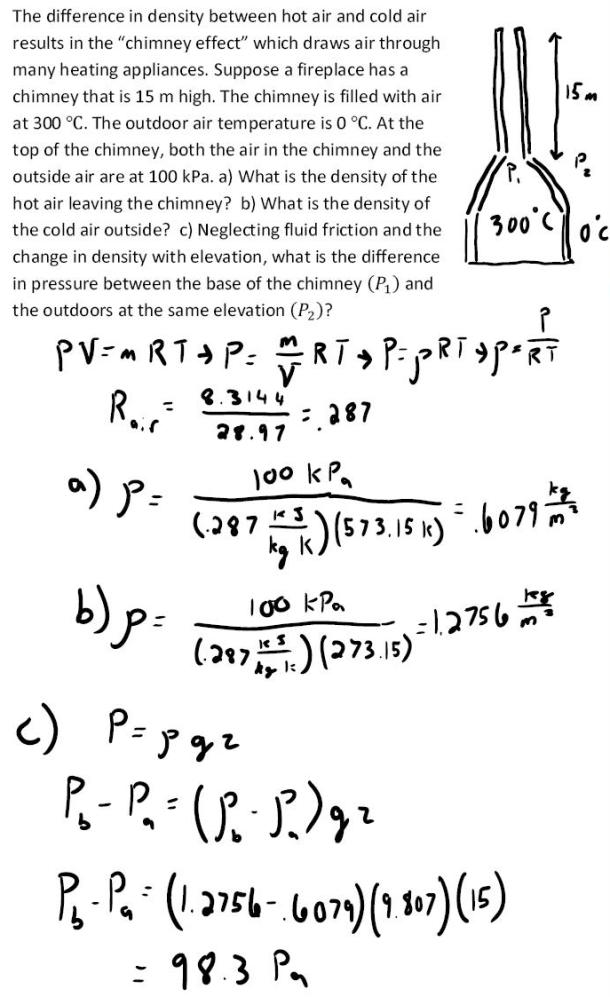fluid mechanics math problems engineering equations fluid mechanics basic mechanics school homework engineering math fluids formulas fluid mechanics problems fluid mechanics problem solutions to fluids problems full solution fluid mechanics engineering fluids problem solution fluid mechanics math problems engineering equations fluid mechanics basic mechanics school homework engineering math fluid mechanics formulas fluid problems fluid mechanics problem solutions to fluid mechanics problems full solution fluid mechanics engineering fluid mechanics problem solution
fluid mechanics math problems engineering equations fluid mechanics basic mechanics school homework engineering math fluids formulas fluid mechanics problems fluid mechanics problem solutions to fluids problems full solution fluid mechanics engineering fluids problem solution fluid mechanics math problems engineering equations fluid mechanics basic mechanics school homework engineering math fluid mechanics formulas fluid problems fluid mechanics problem solutions to fluid mechanics problems full solution fluid mechanics engineering fluid mechanics problem solution
Highalphabet Home Page fluid mechanics solutions fluids math solved Fluid Mechanics Page 1
The difference in density between hot air and cold air results in the chimney effect which draws air through many heating appliances. Suppose a fireplace has a chimney that is 15 m high. The chimney is filled with air at 300 degree C. the outdoor air temperature is 0 degrees C. At the top of the chimney, both the air in the chimney and the outside air are at 100 kPa. a) What is the density of the hot air leaving the chimney? b) What is the density of the cold air outside? c) Neglecting fluid friction and the change in density with elevation, what is the difference in pressure between the base of the chimney and the outdoors at the same elevation?The difference in density between hot air and cold air results in the chimney effect which draws air through many heating appliances. Suppose a fireplace has a chimney that is 15 m high. The chimney is filled with air at 300 degree C. the outdoor air temperature is 0 degrees C. At the top of the chimney, both the air in the chimney and the outside air are at 100 kPa. a) What is the density of the hot air leaving the chimney? b) What is the density of the cold air outside? c) Neglecting fluid friction and the change in density with elevation, what is the difference in pressure between the base of the chimney and the outdoors at the same elevation?